Chapter 21 Electric Charge and Electric Field Power

• Slides: 31Chapter 21 Electric Charge and Electric Field Power. Point® Lectures for University Physics, Thirteenth Edition – Hugh D. Young and Roger A. Freedman Lectures by Wayne Anderson Copyright © 2012 Pearson Education Inc.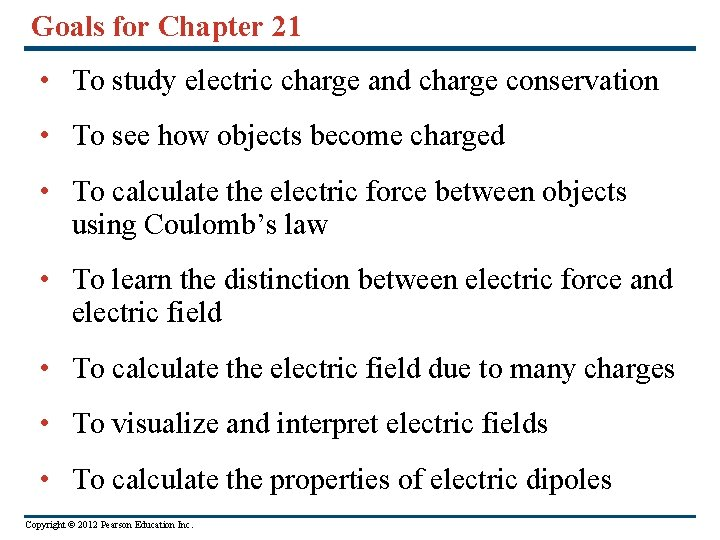Goals for Chapter 21 • To study electric charge and charge conservation • To see how objects become charged • To calculate the electric force between objects using Coulomb’s law • To learn the distinction between electric force and electric field • To calculate the electric field due to many charges • To visualize and interpret electric fields • To calculate the properties of electric dipoles Copyright © 2012 Pearson Education Inc.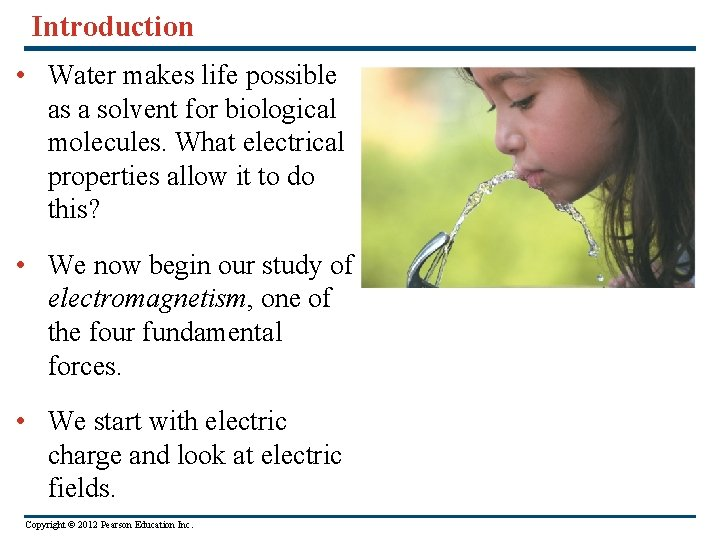Introduction • Water makes life possible as a solvent for biological molecules. What electrical properties allow it to do this? • We now begin our study of electromagnetism, one of the four fundamental forces. • We start with electric charge and look at electric fields. Copyright © 2012 Pearson Education Inc.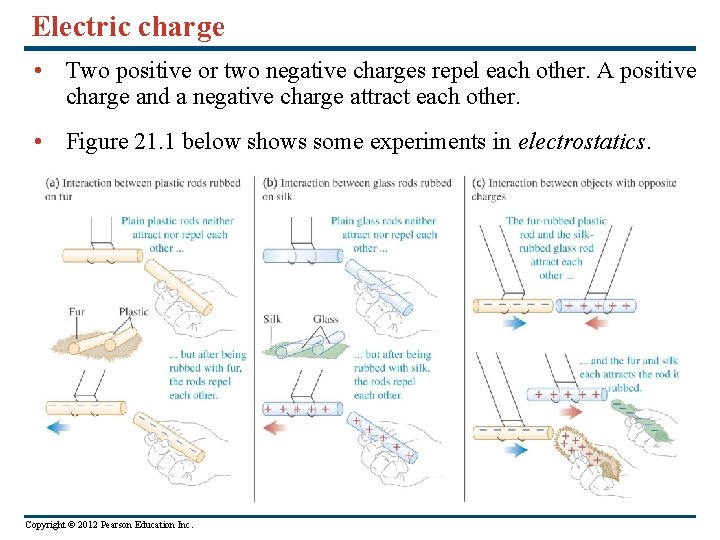Electric charge • Two positive or two negative charges repel each other. A positive charge and a negative charge attract each other. • Figure 21. 1 below shows some experiments in electrostatics. Copyright © 2012 Pearson Education Inc.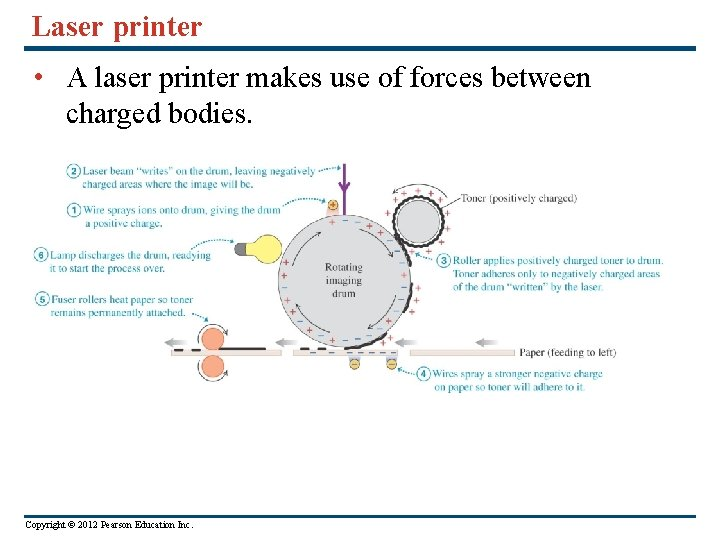Laser printer • A laser printer makes use of forces between charged bodies. Copyright © 2012 Pearson Education Inc.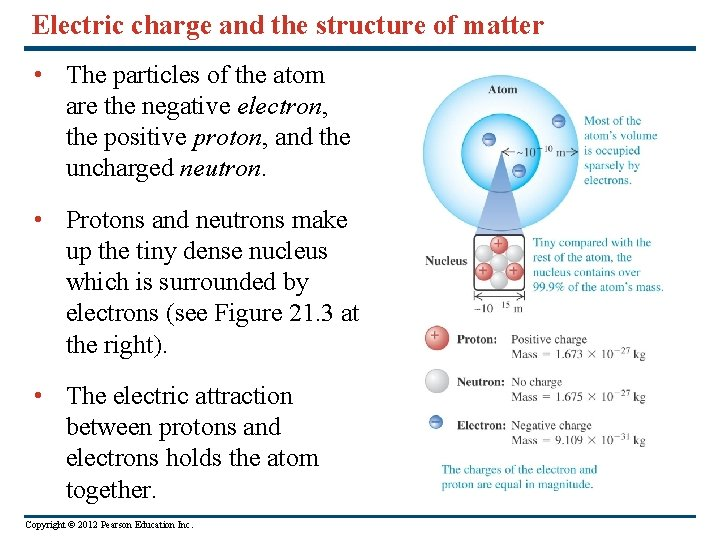Electric charge and the structure of matter • The particles of the atom are the negative electron, the positive proton, and the uncharged neutron. • Protons and neutrons make up the tiny dense nucleus which is surrounded by electrons (see Figure 21. 3 at the right). • The electric attraction between protons and electrons holds the atom together. Copyright © 2012 Pearson Education Inc.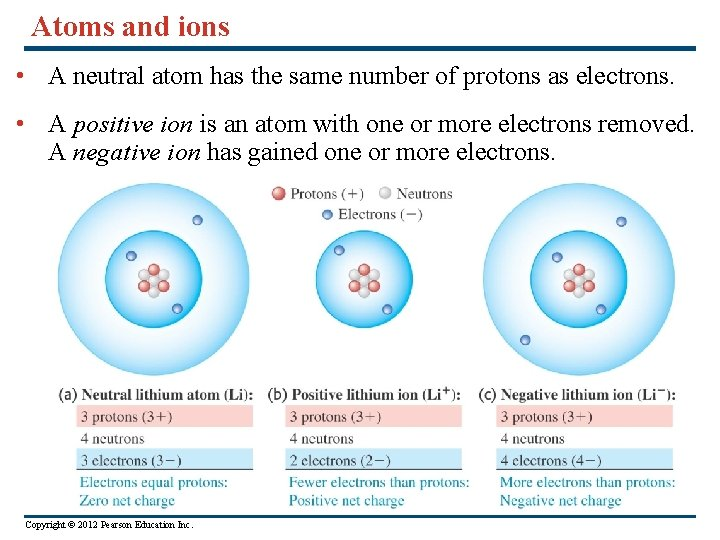Atoms and ions • A neutral atom has the same number of protons as electrons. • A positive ion is an atom with one or more electrons removed. A negative ion has gained one or more electrons. Copyright © 2012 Pearson Education Inc.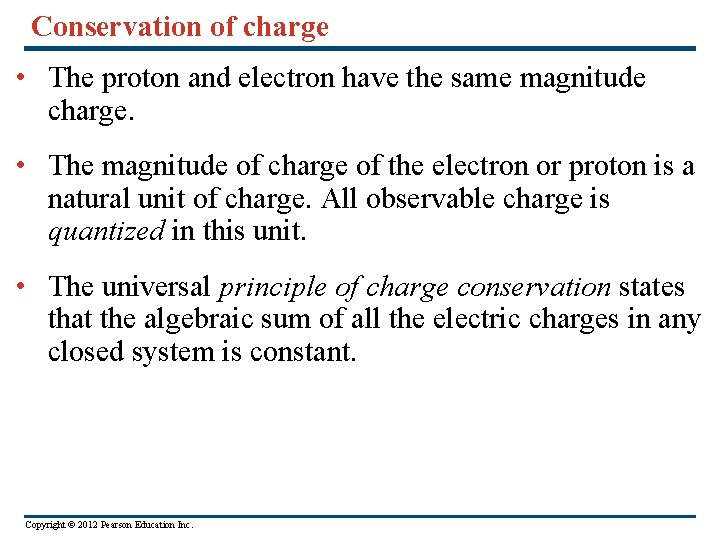Conservation of charge • The proton and electron have the same magnitude charge. • The magnitude of charge of the electron or proton is a natural unit of charge. All observable charge is quantized in this unit. • The universal principle of charge conservation states that the algebraic sum of all the electric charges in any closed system is constant. Copyright © 2012 Pearson Education Inc.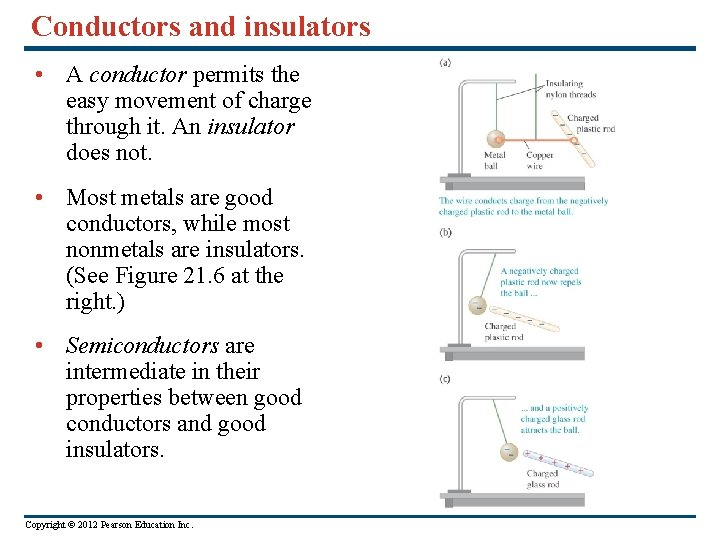Conductors and insulators • A conductor permits the easy movement of charge through it. An insulator does not. • Most metals are good conductors, while most nonmetals are insulators. (See Figure 21. 6 at the right. ) • Semiconductors are intermediate in their properties between good conductors and good insulators. Copyright © 2012 Pearson Education Inc.Charging by induction • In Figure 21. 7 below, the negative rod is able to charge the metal ball without losing any of its own charge. This process is called charging by induction. Copyright © 2012 Pearson Education Inc.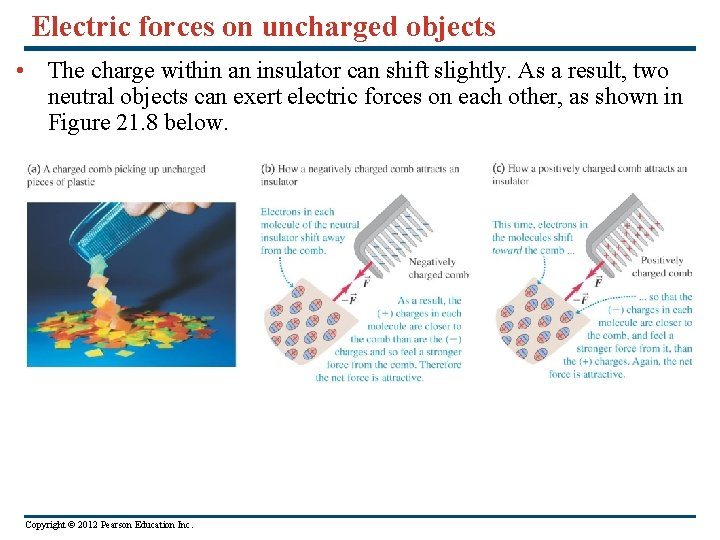Electric forces on uncharged objects • The charge within an insulator can shift slightly. As a result, two neutral objects can exert electric forces on each other, as shown in Figure 21. 8 below. Copyright © 2012 Pearson Education Inc.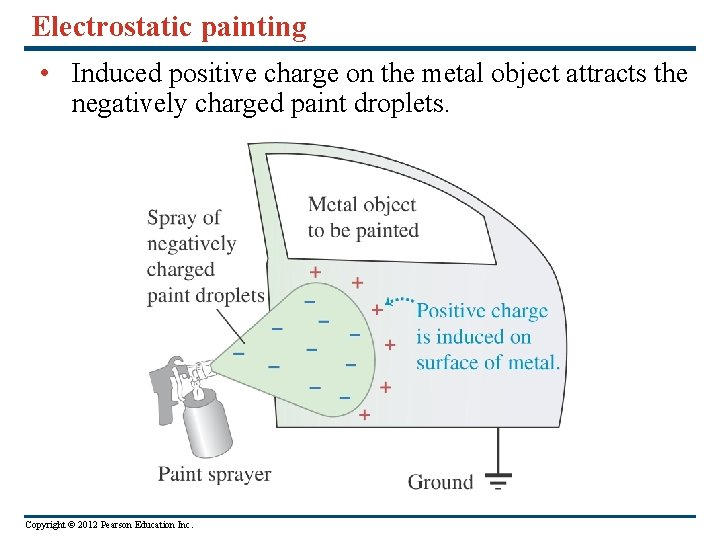Electrostatic painting • Induced positive charge on the metal object attracts the negatively charged paint droplets. Copyright © 2012 Pearson Education Inc.Coulomb’s law • Coulomb’s Law: The magnitude of the electric force between two point charges is directly proportional to the product of their charges and inversely proportional to the square of the distance between them. (See the figure at the right. ) • Mathematically: F = k|q 1 q 2|/r 2 = (1/4π 0)|q 1 q 2|/r 2 Copyright © 2012 Pearson Education Inc.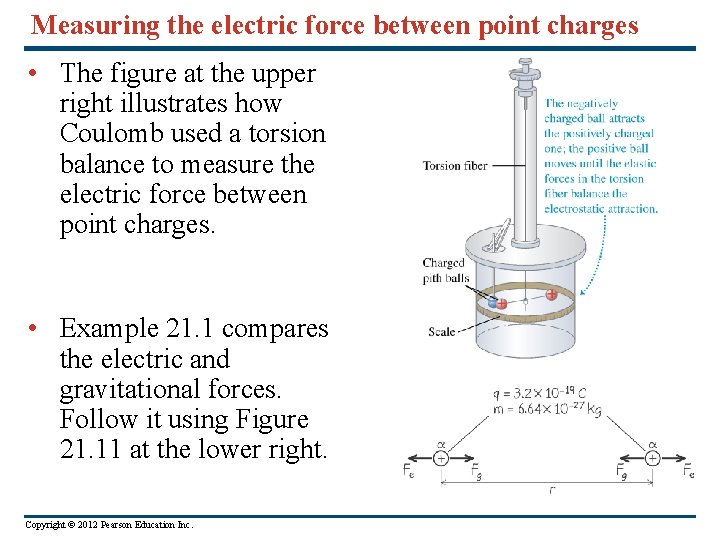Measuring the electric force between point charges • The figure at the upper right illustrates how Coulomb used a torsion balance to measure the electric force between point charges. • Example 21. 1 compares the electric and gravitational forces. Follow it using Figure 21. 11 at the lower right. Copyright © 2012 Pearson Education Inc.Force between charges along a line • Read Problem-Solving Strategy 21. 1. • Follow Example 21. 2 for two charges, using Figure 21. 12 at the right. • Follow Example 21. 3 for three charges, using Figure 21. 13 below. Copyright © 2012 Pearson Education Inc.Vector addition of electric forces • Example 21. 4 shows that we must use vector addition when adding electric forces. Follow this example using Figure 21. 14 below. Copyright © 2012 Pearson Education Inc.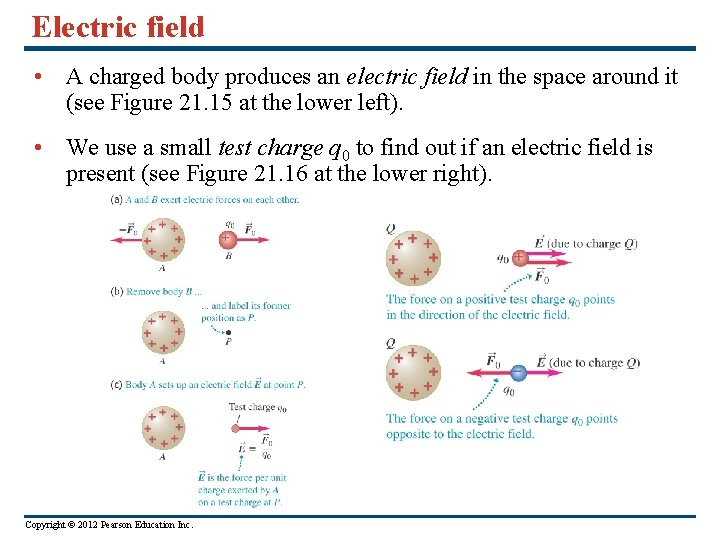Electric field • A charged body produces an electric field in the space around it (see Figure 21. 15 at the lower left). • We use a small test charge q 0 to find out if an electric field is present (see Figure 21. 16 at the lower right). Copyright © 2012 Pearson Education Inc.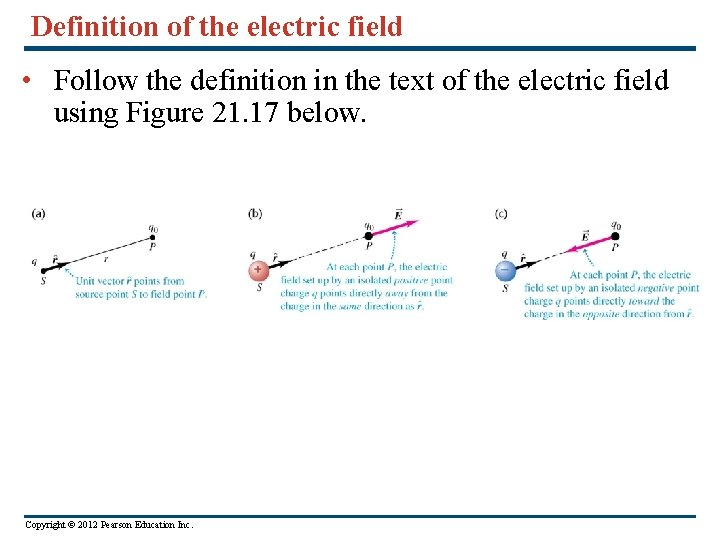Definition of the electric field • Follow the definition in the text of the electric field using Figure 21. 17 below. Copyright © 2012 Pearson Education Inc.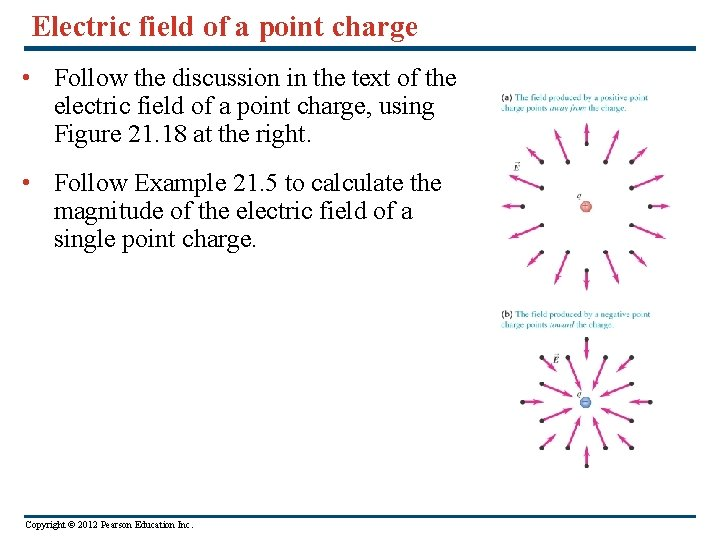Electric field of a point charge • Follow the discussion in the text of the electric field of a point charge, using Figure 21. 18 at the right. • Follow Example 21. 5 to calculate the magnitude of the electric field of a single point charge. Copyright © 2012 Pearson Education Inc.Electric-field vector of a point charge • Follow Example 21. 6 to see the vector nature of the electric field. Use Figure 21. 19 at the right. Copyright © 2012 Pearson Education Inc.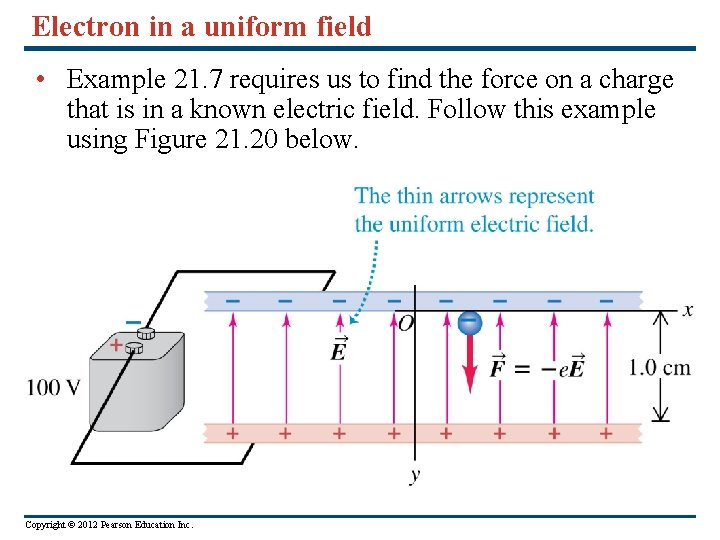Electron in a uniform field • Example 21. 7 requires us to find the force on a charge that is in a known electric field. Follow this example using Figure 21. 20 below. Copyright © 2012 Pearson Education Inc.Superposition of electric fields • The total electric field at a point is the vector sum of the fields due to all the charges present. (See Figure 21. 21 below right. ) • Review Problem-Solving Strategy 21. 2. • Follow Example 21. 8 for an electric dipole. Use Figure 21. 22 below. Copyright © 2012 Pearson Education Inc.Field of a ring of charge • Follow Example 21. 9 using Figure 21. 23 below. Copyright © 2012 Pearson Education Inc.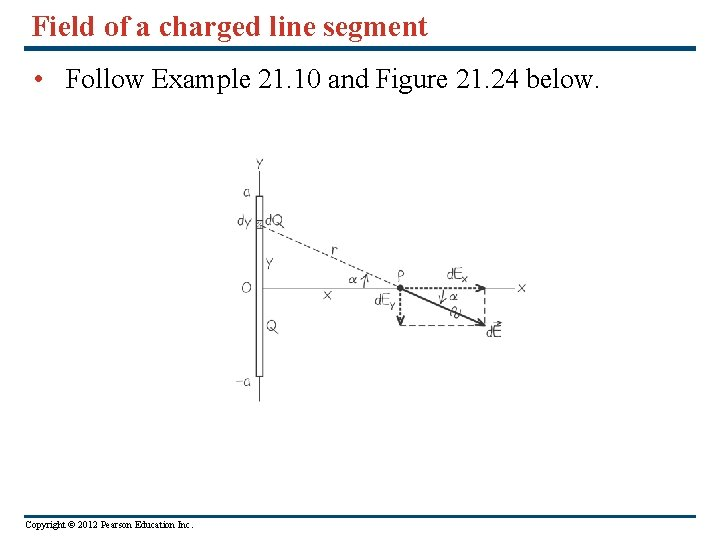Field of a charged line segment • Follow Example 21. 10 and Figure 21. 24 below. Copyright © 2012 Pearson Education Inc.Field of a uniformly charged disk • Follow Example 21. 11 using Figure 21. 25 below. Copyright © 2012 Pearson Education Inc.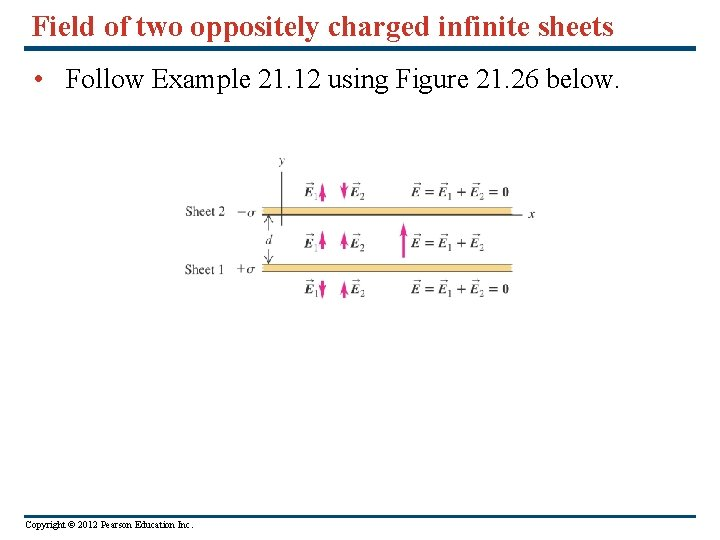Field of two oppositely charged infinite sheets • Follow Example 21. 12 using Figure 21. 26 below. Copyright © 2012 Pearson Education Inc.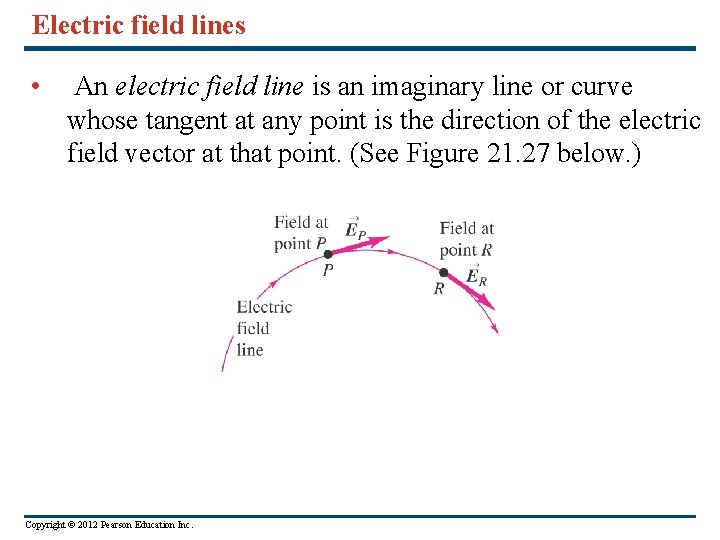Electric field lines • An electric field line is an imaginary line or curve whose tangent at any point is the direction of the electric field vector at that point. (See Figure 21. 27 below. ) Copyright © 2012 Pearson Education Inc.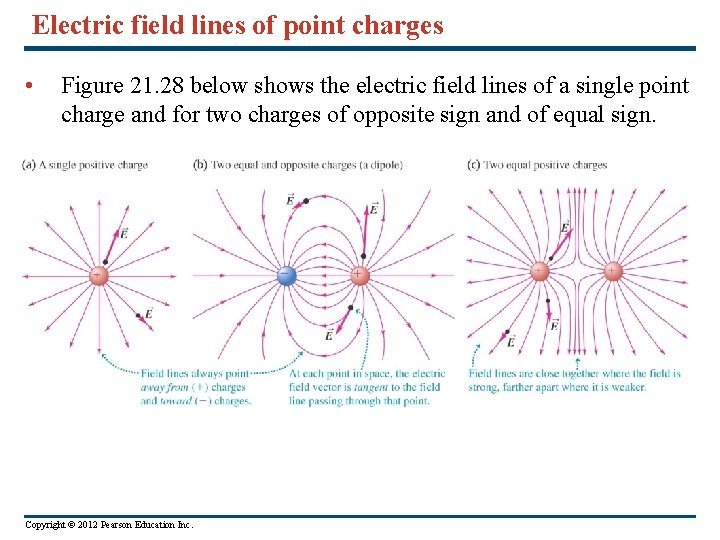Electric field lines of point charges • Figure 21. 28 below shows the electric field lines of a single point charge and for two charges of opposite sign and of equal sign. Copyright © 2012 Pearson Education Inc.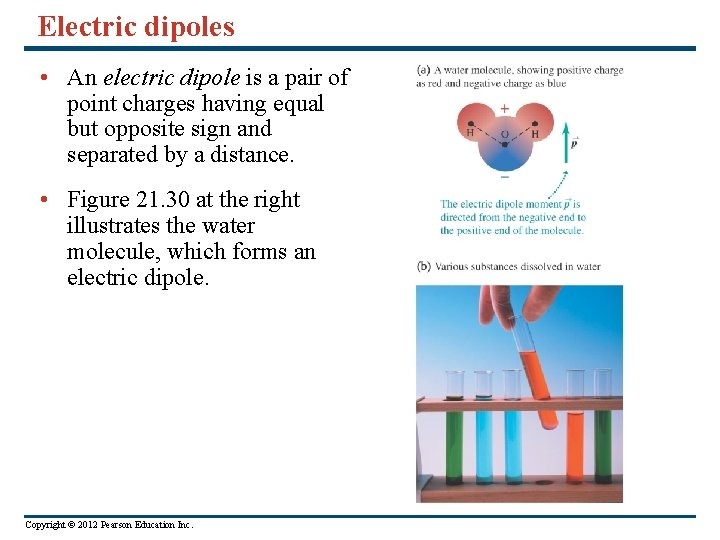Electric dipoles • An electric dipole is a pair of point charges having equal but opposite sign and separated by a distance. • Figure 21. 30 at the right illustrates the water molecule, which forms an electric dipole. Copyright © 2012 Pearson Education Inc.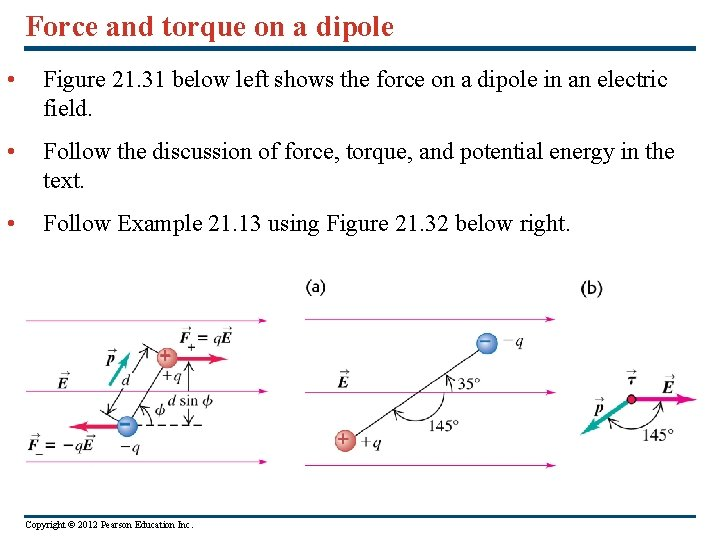Force and torque on a dipole • Figure 21. 31 below left shows the force on a dipole in an electric field. • Follow the discussion of force, torque, and potential energy in the text. • Follow Example 21. 13 using Figure 21. 32 below right. Copyright © 2012 Pearson Education Inc.Electric field of a dipole • Follow Example 21. 14 using Figure 21. 33. Copyright © 2012 Pearson Education Inc.